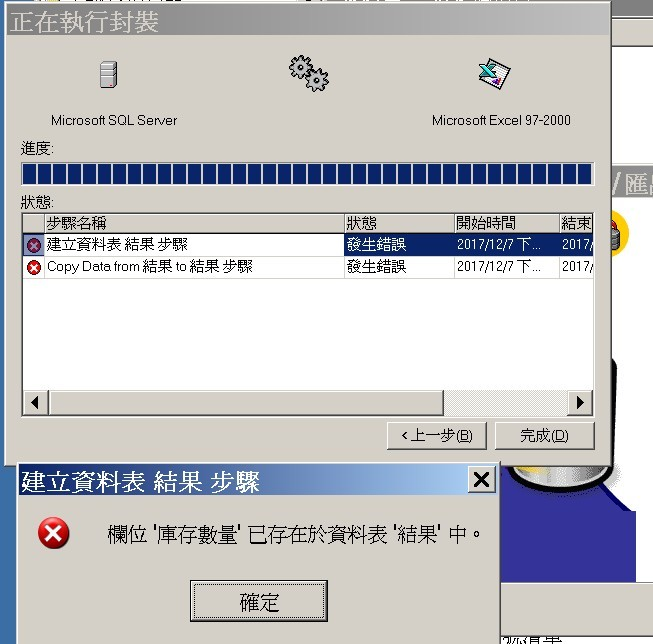#0

## MS SQL2000查詢&剖析語法正確，但匯出EXCEL檔失敗

Dear All---1
Select a.商品貨號, a.商品名稱, b.庫存數量, c.庫存數量, d.庫存數量 from 商品基本檔 a
Inner join (select * from 商品庫存檔 where 門市編號='1001') b on a.商品貨號=b.商品貨號
Inner join (select * from 商品庫存檔 where 門市編號='1003') c on a.商品貨號=c.商品貨號
Inner join (select * from 商品庫存檔 where 門市編號='1005') d on a.商品貨號=d.商品貨號
Where a.大類編號='8' And (b.庫存數量 < = 0 And c.庫存數量 > = 0 And d.庫存數量 >= 1)
Order by a.商品貨號
---2
Select a.商品貨號, a.商品名稱, b.庫存數量, c.庫存數量, d.庫存數量 from 商品基本檔 a
Inner join (select 商品貨號, 庫存數量 from 商品庫存檔 where 門市編號='1001') b on a.商品貨號=b.商品貨號
Inner join (select 商品貨號, 庫存數量 from 商品庫存檔 where 門市編號='1003') c on a.商品貨號=c.商品貨號
Inner join (select 商品貨號, 庫存數量 from 商品庫存檔 where 門市編號='1005') d on a.商品貨號=d.商品貨號
Where a.大類編號='8' And (b.庫存數量 < = 0 And c.庫存數量 > = 0 And d.庫存數量 >= 1)
Order by a.商品貨號
---3
Select a.商品貨號, a.商品名稱, b.庫存數量, c.庫存數量, d.庫存數量 from 商品基本檔 a
Inner join (Select 商品貨號 as b貨號, 庫存數量 from 商品庫存檔 where 門市編號='1001') b on a.商品貨號=b.b貨號
Inner join (Select 商品貨號 as c貨號, 庫存數量 from 商品庫存檔 where 門市編號='1003') c on a.商品貨號=c.c貨號
Inner join (Select 商品貨號 as d貨號, 庫存數量 from 商品庫存檔 where 門市編號='1005') d on a.商品貨號=d.d貨號
Where a.大類編號='8' And (b.庫存數量 < = 0 And c.庫存數量 > = 0 And d.庫存數量 >= 1)
Order by a.商品貨號

### 2 個回答

1

sjzgo iT邦新手 4 級 ‧ 2017-12-07 23:02:38 檢舉sjzgo iT邦新手 4 級 ‧ 2017-12-07 23:04:33 檢舉

``````Select a.商品貨號, a.商品名稱, b.庫存數量 AS 庫存數量1001, c.庫存數量 AS 庫存數量1003, d.庫存數量 AS 庫存數量1005 from 商品基本檔 a
Inner join (select * from 商品庫存檔 where 門市編號='1001') b on a.商品貨號=b.商品貨號
Inner join (select * from 商品庫存檔 where 門市編號='1003') c on a.商品貨號=c.商品貨號
Inner join (select * from 商品庫存檔 where 門市編號='1005') d on a.商品貨號=d.商品貨號
Where a.大類編號='8' And (b.庫存數量 < = 0 And c.庫存數量 > = 0 And d.庫存數量 >= 1)
Order by a.商品貨號
``````
sjzgo iT邦新手 4 級 ‧ 2017-12-08 11:28:25 檢舉

sjzgo iT邦新手 4 級 ‧ 2017-12-08 12:47:54 檢舉